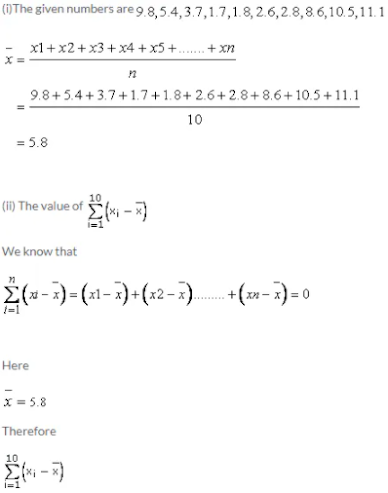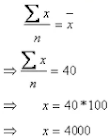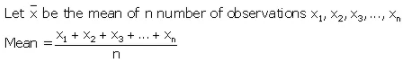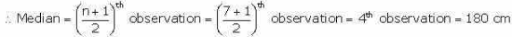# ICSE Solutions for Selina Concise Chapter 19 Mean and Median Class 9 Maths

### Exercise 19(A)

1. Find the mean of 43, 51, 50, 57 and 54.

The numbers given are 43, 51, 50, 57, 54
The mean of the given numbers will be
= (43+51+50+57+54)/5
= 255/5
= 51

2. Find the mean of first six natural numbers.

The first six natural number are 1, 2 ,3, 4, 5, 6
The man of first six natural numbers
= (1+2+3+4+5+6)/3
= 21/3
= 7

3. Find the mean of first ten odd natural number.

The first ten odd natural numbers are 1,3,5,7,9,11,13,15,17,19
The mean of first ten odd numbers
=(1+3+5+7+9+11+13+15+17+19)/10
= 100/10
= 10

4. Find the mean of all factors of 10.

The all factors of 10 are 1,2,5,10
The mean of all factors of 10 are
= (1+2+5+10)/4
= 18/4
= 4.5

5. Find the mean of x + 3, x + 5, x + 7, x + 9 and x + 11.

The given values are x+3, x+5, x+7, x+9,x+11
The mean of the values are
= (x+3+x+5+x+7+x+9+x+11)/5
= (5x+35)/5
= 5(x+7)/5
= x+7

6. If different values of variable x are 9.8, 5.4, 3.7, 1.7, 1.8, 2.6, 2.8, 8.6, 10.5 and 11.1; find= (9.8-5.8) + (5.4-5.8) + (3.7-5.8) + (1.7-5.8) + (1.8-5.8) + (2.6-5.8) + (2.8-5.8) + (8.6-5.8) + (10.5-5.8) + (11.1-5.8)
= 4 - 4 - 2.1 - 4.1 - 4 - 3.2 - 3 + 2.8 + 4.7 + 5.3
= 0

7. The mean of 15 observations is 32. Find the resulting mean, if each observation is :

(i) Increased by 3

(ii) Decreased by 7

(iii) Multiplied by 2

(iv) Divided by 0.5

(v) Increased by 60%

(vi) Decreased by 20%

Given that the mean of 15 observations  is 32
(i) resulting mean increased by 3 = 32+ 3 = 35
(ii) resulting mean decreased by 7 = 32 - 7 = 25
(iii) resulting mean multiplied by 2 = 32×2 = 64
(iv) resulting mean divide by 0.5 = 32/0.5 = 64
(v) resulting mean increased by 60%
= 32 + (60/100)×32
= 32 + 19.2
= 51.2
(vi) resulting mean decreased by 20%
= 32- (20/100)×32
= 32 - 6.4
= 25.6

8. The mean of 5 numbers is 18. If one number is excluded, the mean of remaining number becomes 16. Find the excluded number.

Given the mean of 5 number is 18
Total sum of 5 numbers
= 18×5
= 90
On excluding an observation, the mean of remaining 4 observation is 16
= 16×4
= 64
Therefore sum of remaining 4 observations
= total of 5 observations - total of 4 observations
= 90 - 64
= 26

9. If the mean of observations x, x + 2, x + 4, x + 6 and x + 8 is 11, find:

(i) The value of x;

(ii) The mean of first three observations.

(i) Given that the mean of observations x, x+2 , x+4, x+6, and x+8 is 11
Mean = observations/n
11 = (x+x+2+x+4+x+6+x+8)/5
⇒ 11 = (5x+20)/5
⇒ x = 35/7
⇒ x = 7
(ii) The mean of first three observations are
= (x+x+2+x+4)/3
= (3x+6)/3
= (3×7 + 6)/3  [since x = 7]
= (21+6)/3
= 9

10. The mean of 100 observations is 40. It is found that an observation 53 was misread as 83. Find the correct mean.

Given the mean of 100 observations is 40.Incorrect value of x = 4000
Correct value of x = Incorrect value of x - Incorrect observation + correct observation
= 4000 - 83 + 53
= 3970
Correct mean
= (correct value of ∑x)/n
= 3970/100
= 39.7

11. The mean of 200 items was 50. Later on, it was discovered that two items were misread as 92 and 8 instead of 192 and 88. Find the correct mean.

Given that the mean of 200 items was 50.
Mean = ∑x/n
⇒ 50 = ∑x/200
⇒ x = 10000
Incorrect value of ∑x = 10000
Correct value of
∑x = 10000 - (92 + 8)+ (192+88)
= 10000 - 100 + 280
= 10180
Correct mean
= (correct value of ∑x)/2
= 10180/200
= 50.9

12. Find the mean of 75 numbers, if the mean of 45 of them is 18 and the mean of the remaining ones is 13.

Mean of 45 numbers = 18
⇒ Sum of 45 numbers = 18×45 = 810
Mean of remaining (75 - 45)30 numbers = 13
⇒ Sum of remaining 30 numbers = 13×30 = 390
⇒ Sum of all the 75 numbers = 810+ 390 = 1200
⇒ Mean of all the 75 numbers = 1200/75 = 16

13. The mean weight of 120 students of a school is 52.75 kg. If the mean weight of 50 of them is 51 kg, find the mean weight of the remaining students.

Mean weight of 120 students = 52.75 kg
⇒ Sum of the weight of 120 students = 120×52.75 = 6330 kg
Mean weight of 50 students = 51 kg
⇒ Sum of the weight of  50 students = 50 ×51 = 2550 kg
⇒ Sum of the weight of remaining (120 - 50) = 70 students
= Sum of the weight of 120 students - Sum of the weight of 50 students
= (6330 - 2550)kg
= 3780 kg
⇒ Mean weight of remaining 70 students = 3780/70 = 54kg

14. The mean marks (out of 100) of boys and girls in an examination are 70 and 73 respectively. If the mean marks of all the students in that examination is 71, find the ratio of the number of boys to the number of girls.

Let the number of boys and girls be x and y respectively.
Now,
Given, Mean marks of x boys in the examination = 70
⇒ Sum of marks of x boys in the examination = 70x
Given, Mean marks of Y girls in the examination = 73
⇒ Sum of marks of Y girls in the examination = 73Y
Given, Mean marks of all students (x+ y) in the examination = 71
⇒ Sum of marks of all students(x+ y) students in the examination = 71(x+y)
Now, Sum of marks of all students (x+ y) students in the examination
= Sum of marks of x boys in the examination + Sum of marks of y girls in the examination
⇒ 71(x+ y) = 70x + 73y
⇒ 71x + 71y = 70x + 73y
⇒ x = 2y
⇒ x/y = 2/1
⇒ x : y = 2: 1
Thus, the ratio of number of boys to the number of girls is 2 : 1.

15. Find x if 9, x, 14, 18 x, x, 8, 10 and 4 have a mean of 11.

Mean = (Sum of observations)/(Total number of observations)
⇒ 11 = (9+x+14+18+x+x+8+10+4)/9
⇒ 11×9 = 63+3x
⇒ 3x = 99-63
⇒ 3x = 36
⇒  x = 12

16. In a series of tests, A appeared for 8 tests. Each test was marked out of 30 and averages 25. However, while checking his files, A could only find 7 of the 8 tests. For these he scored 29, 26, 18, 20, 27, 24 and 29. Determine how many marks he scored for the eighth test.

Total number of tests = 8

Average score of A = 25

Let the score of 8th test be x.

Then, total score of 8 tests = 29 + 26 + 18 + 20 + 27 + 24 + 29 + x

Now, we have

Mean = (Total score of 8 tests)/(Total number of tests)
⇒ 25 = (29+26+18+20+27+24+29+x)/8

⇒ 25×8 =173 + x

⇒ x +173 = 200

⇒ x = 200 – 173

⇒ x = 27

Thus, A scored 27 marks in the eights test.

### Exercise 19(B)

1. Find the median of:

(i) 25, 16, 26, 16, 32, 31, 19, 28 and 35

(ii) 241, 243, 347, 350, 327, 299, 261, 292, 271, 258 and 257

(iii) 63, 17, 50, 9, 25, 43, 21, 50, 14 and 34

(iv) 233, 173, 189, 208, 194, 204, 194, 185, 200 and 220.

(i) Firstly arrange the numbers in ascending order
16,16,19,25,26,28,3132,35
Now since
n = 9(odd)
Therefore Median

(ii)  Firstly arrange the numbers in ascending order
241,243,257,258,261,271,292,299,327,347,350,
Now since n = 11(Odd)

2. The following data have been arranged in ascending order. If their median is 63, find the value of x.

34, 37, 53, 55, x, x + 2, 77, 83, 89 and 100.

Given numbers are 34, 37,53,55,x,x+2,77,83,89,100
Here n = 10(even)

3. In 10 numbers, arranged in increasing order, the 7th number is increased by 8, how much will the median be changed?

For any given set of data, the median is the value of its middle term.
Here, total observations = n = 10(even)
If n is even, we have

4. Out of 10 students, who appeared in a test, three secured less than 30 marks and 3 secured more than 75 marks. The marks secured by the remaining 4 students are 35, 48, 66 and 40. Find the median score of the whole group.

Here, total observations = n = 10(even)
Thus, we have

5. The median of observations 10, 11, 13, 17, x + 5, 20, 22, 24 and 53 (arranged in ascending order) is 18; find the value of x.

Total number of observations = 9(odd)
Now, if n = odd

### Exercise -19(C)

1. Find the mean of 8, 12, 16, 22, 10 and 4. Find the resulting mean, if each of the observations, given above, be:

(i) multiplied by 3.

(ii) divided by 2.

(iii) multiplied by 3 and then divided by 2.

(iv) increased by 25%

(v) decreased by 40%

Mean of the given data = (8+12+16+22+10+4)/6
= 72/6 = 12
(i) Multiplied by 3Thus, when each of the given data is multiplied by 3,
the mean is also multiplied by  3.
Mean of the original data is 12.
Hence, the new mean = 12×3 = 36.

(ii) Divided by  2Thus, when each of the given data is divided by 2,
the mean is also divided by  3.
Mean of the original data is 12.
Hence, the new mean = 12/2 = 6.
(iii) multiplied by 3 and then divided by  2Thus, when each of the given data is multiplied by 3/2,
the mean is also multiplied by 3/2.
Mean of the original data is 12.
Hence, the new mean = (3/2)×12 = 36/2 = 18
(iv) increased by 25%
New mean = Original mean + 25% of original mean
⇒ New mean = 12 +25% of  12
⇒ New mean = 12 + (25/100)×12
⇒ New mean = 12 + (1/4)×12
⇒ New mean = 12 +3
⇒ New mean = 15
(v) decreased by  40%
New mean = Original mean - 40% of original mean
⇒ New mean = 12 - 40% of  12
⇒ New mean = 12 - (40/100)×12
⇒ New mean = 12 - (2/5)×12
⇒ New mean = 12 - 0.4×12
⇒ New mean = 12 - 4.8
⇒ New mean = 7.2

2. The mean of 18, 24, 15, 2x + 1 and 12 is 21. Find the value of x.

Mean of given data = (18 + 24+15+2x+1+12)/5
⇒ 21 = (70 + 2x)/5
⇒ 5×21 = 70 +2x
⇒ 105 = 70 +2x
⇒ 2x = 105- 70
⇒ 2x = 35
⇒ x = 35/2
⇒ x = 17.5

3. The mean of 6 numbers is 42. If one number is excluded, the mean of remaining number is 45. Find the excluded number.

4. The mean of 10 numbers is 24. If one more number is included, the new mean is 25. Find the included number.

5. The following observations have been arranged in ascending order. If the median of the data is 78, find the value of x.

44, 47, 63, 65, x + 13, 87, 93, 99, 110.

Consider the given data :
44,47,63,65,x+13,87,93,99,110
Here the number of observations is 9, which is odd.
Thus, the median of the given data is (n+1/2)th observation.
From the given data,[(9+1)/2 = 5th observation is x + 13
Also, given that the median is 78.
Thus, we have
x+ 13 = 78
⇒ x = 78 - 13
⇒ x = 65

6. The following observations have been arranged in ascending order. If the median of these observations is 58, find the value of x.

24, 27, 43, 48, x – 1, x + 3, 68, 73, 80, 90.

Consider the given data :
24, 27, 43, 48, x-1, x+3, 68, 73, 80, 90.
Here the number of observations is 10, which is even.

7. Find the mean of the following data:

30, 32, 24, 34, 26, 28, 30, 35, 33, 25

(i) Show that the sum of the deviations of all the given observation from the mean is zero.

(ii) Find the median of the given data.

(iii) Consider the given data :
30,32,24,34,26,28,30,35,33,25
Let us rewrite the above data in ascending order.
24,25,26,28,30,30,32,33,34,35,
There are 10 observations which is even.

8. Find the mean and median of the data:

35, 48, 92, 76, 64, 52, 51, 63 and 71.

If 51 is replaced by 66, what will be the new median?Therefore,
Mean of given data = (35+48+92+76+64+52+51+63+71)/9
= 552/9
= 61.33
Let us rewrite the given data in ascending order :
Thus, we have
35,48,51,52,63,64,71,76,92,
There are 9 observations, which is odd.
Therefore, median = [(n+1)/2]th  observation
⇒ Median = [(9+1)/2]th observation
⇒ Median = (10/2)th observation
⇒ Median = 5th observation
⇒ Median = 63
If 51 is replaced by 66, the new set of data in ascending order is :
35,48,52,63,64,66,71,76,92,
Since median = 5th observation,
We have, new median = 64

9. The mean of x, x + 2, x + 4, x + 6 and x + 8 is 11, find the mean of the first three observations.Therefore,
Mean of given data = (x+x+2+x+4+x+6+x+8)/5
= (5x + 20)/5
= x + 4
Also, it's given that mean of the given data is 11 .
⇒ x+ 4 = 11
⇒ x =7
Hence the mean of the first three observations = (x+x+2+x+4)/3
= (3x+6)/3
= x+ 2
= 7+2
= 9

10. Find the mean and median of all the positive factors of 72.

Let us find the factors of 72 :
72 = 1×72
= 2×36
= 3×24
= 4×18
= 6×12
= 8×9
= 9×8
= 12×6
= 18×4
= 24×3
= 36×2
= 72×1
Therefore, the data set is :
1,2,3,4,6,8,9,12,18,24,36,72
Mean of the above data set = (1+2+3+4+6+8+9+12+18+24+36+72)/12
= 195/12
= 16.25
Since the number of observations is 12, which is even,
median is given by

11. The mean weight of 60 students in a class is 40 kg. The mean weight of boys is 50 kg while that of girls is 30 kg. Find the number of boys and girls in the class.

Total number of students = 60

Mean weight of 60 students = 40

Let the number of boys = x

Then, number of girls = 60 – x

Mean weight of boys = (Total weight of boys)/(Total number of boys)
⇒ 50 = (Total weight of boys)/x
⇒ Total weight of boys = 50x
Mean weight of girls = (Total weight of girls)/(Total number of girls)
⇒ 30 = (Total weight of girls)/(60-x)
⇒ Total weight of girls = 30(60 -x )
Now,
Mean weight of 60 students = (Total weight of boys + Total weight of girls)/Total number of students
⇒ 40 = [50x + 30(60-x)]/60
⇒ 2400 = 50x + 1800 - 30x
⇒ 20x = 600
⇒ x = 30

Hence, the number of boys is 30 and the number of girls is also 30.

12. The average of n numbers x1, x2, x3 ….. xn is A. If x1 is replaced by (x+ α)x1, x2, is replaced by (x+ α)x2 and so on. Find the new average.

Mean of n numbers = A

13. The heights (in cm) of the volley- ball players from team A and team B were recorded as:

Team A: 180, 178, 176, 181, 190, 175, 187

Team B: 174, 175, 190, 179, 178, 185, 177

Which team had the greater average height?

Find the median of team A and team B.

Total number of players in each team = 7
Mean height of team A = (Sum of heights of players of team A)/(Total number of team A players)
= (180+178+176+181+190+175+187)/7
= 1267/7
= 181 cm
Mean height of team B = (Sum of heights of players of team B)/(Total number of team B players)
= (174+175+190+179+178+185+177)/7
= 1258/7
= 179.7cm
Thus, team A has greater average height
Median of team A :
Arranging heights in ascending order, we get
175,176,178,180,181,187,190,
Total number of observations = n = 7(odd)Median of team B :
Arranging heights in ascending order, we get
174,175,177,178,179,185,190
Total number of observations = n = 7(odd)